Kindergarten
1st
2nd
3rd
4th
5th
6th
7th
8th
9th
10th
11th
12th
Higher Ed
Other
Subjects
ELA
Math
Science
Social Studies
Art
Computer Science
French
German
Music
Physical Education
Spanish
Other
Private Library
Surface Area of Cubes and Rectangular Prism Test
starstarstarstarstarstarstarstarstarstar
4 (1 rating)
by Andrew Allen
| 13 Questions
Note from the author:
Surface Area of Cubes and Rectangular Prisms, 3D and Nets1
1 pt
What is the surface area of the figure above?
192
208
2402
1 pt
What is the surface area of the figure above?
30
62
72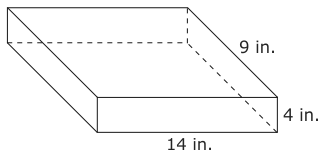3
1 pt
What is the surface area of the figure above?
436
504
5764
1 pt
What is the surface area of the above figure?
95
120
158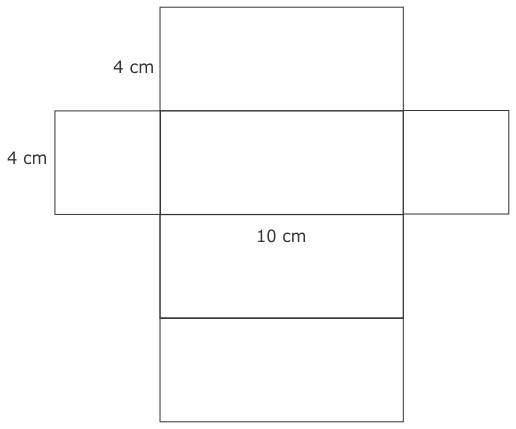5
1 pt
What is the surface area of the figure above?
160
176
192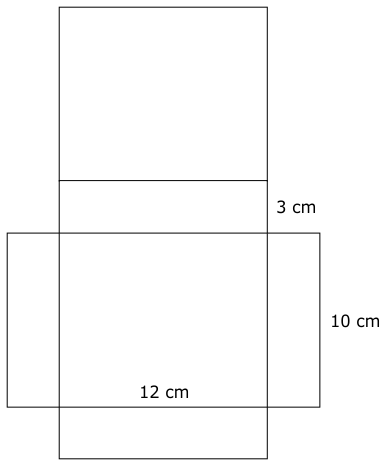6
1 pt
What is the surface area of the figure above?
186
240
360
372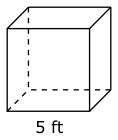7
1 pt
What is the surface area of the figure above?
100
125
1508
1 pt
What is the surface area of the above cube if it has a side length of 8 inches?
512
384
288
96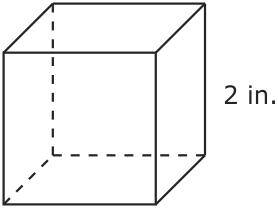9
1 pt
What is the surface area of the figure above?
24
16
12
8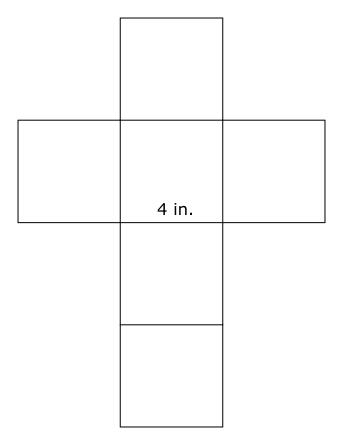10
1 pt
What is the surface area of the figure above?
16
24
64
96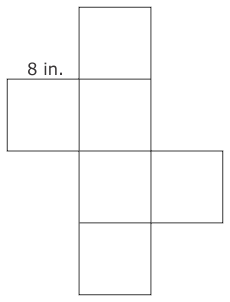11
1 pt
What is the surface area of the figure above?
96
192
384
51212
1 pt
The net of a cube is shown above. The cube has a surface area of 24ft^2. What is the length of the side?
2
4
6
1213
1 pt
Extra Credit, it will only HELP you not hurt you. Find the surface area of the above figure. Remember what surface area is and that will give a clue to how to solve this problem.
Add to my formatives list